# Lecture 14 - Graphs and basic search

Date Pre-lecture slides Post-lecture scribbles Lecture recording
October 12 2023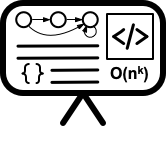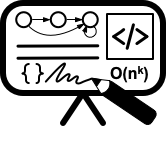## Notes

#### I. Undirected graphs

##### Definition

An undirected (simple) graph G = (V, E) is a 2-tuple:

• V is a set of vertices (also referred to as nodes/points)
• E is a set of edges where each edge e belong to E is a set of the form {u, v} with u, v belong to V and u not equala to v.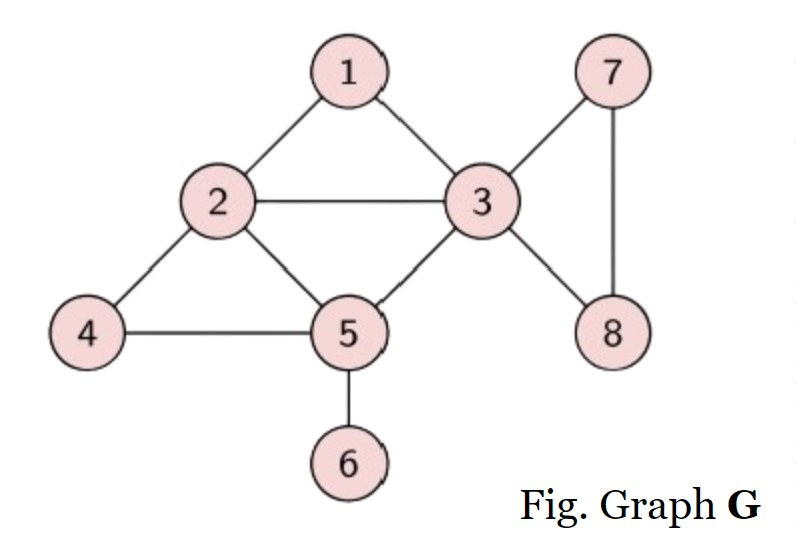Example: In figure, G= (V,E) where V = {1,2,3,4,5,6,7,8} ; E = {(1, 2), (1, 3), (2, 3), (2, 4), (2, 5), (3, 5), (3, 7), (3, 8), (4, 5), (5, 6), (7, 8)}
##### Notation and Convention

An edge in an undirected graphs is an unordered pair of nodes and hence it is a set. Conventionally we use uv for {u, v} when it is clear from the context that the graph is undirected.

• u and v are the end points of an edge {u, v}
• Multi-graphs allow:
• loops which are edges with the same node appearing as both end points
• multi-edges: diferent edges between same pairs of nodes
##### Graph Representation I - Adjacency Matrix

Represent G = (V, E) with n vertices and m edges using a n x n adjacency matrix A where

• A[i, j] = A[j, i] = 1 if {i, j} $\in$ E and A[i, j] = A[j, i] = 0 if {i, j} $\notin$ E.
• Advantage: can check if {i, j} $\in$ E in O(1) time
• Disadvantage: needs $\Omega$ ($n^2$) space even when m « n$^2$

Example: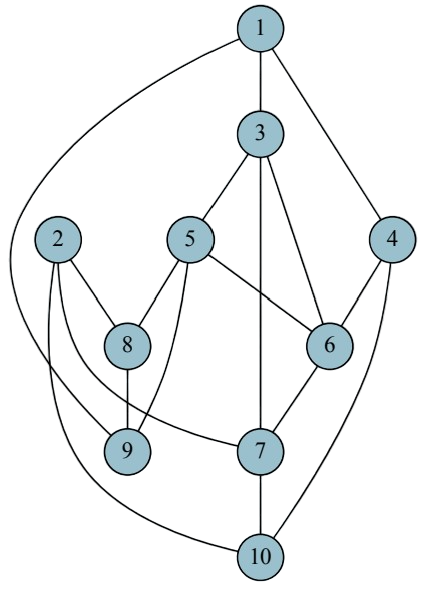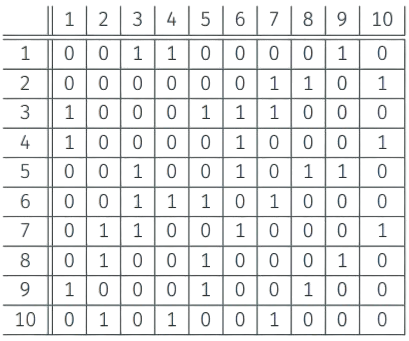##### Graph Representation II - Adjacency List

Represent G = (V, E) with n vertices and m edges using adjacency lists:

•  For each u $\in$ V, Adj(u) = {v {u, v} $\in$ E}, that is neighbors of u. Sometimes Adj(u) is the list of edges incident to u.
• Advantage: space is O(m + n)
• Disadvantage: cannot “easily” determine in O(1) time whether {i, j} $\in$ E
• By sorting each list, one can achieve O(log n) time
• By hashing “appropriately”, one can achieve O(1) time.

Example:##### Concrete Representation

Assume vertices are numbered arbitrarily as {1, 2,…, n}.

• Edges are numbered arbitrarily as {1, 2,…, m}.
• Edges stored in an array/list of size m. E[j] is j$^{th}$ edge with info on end points which are integers in range 1 to n.
• Array Adj of size n for adjacency lists. Adj[i] points to adjacency list of vertex i. Adj[i] is a list of edge indices in range 1 to m.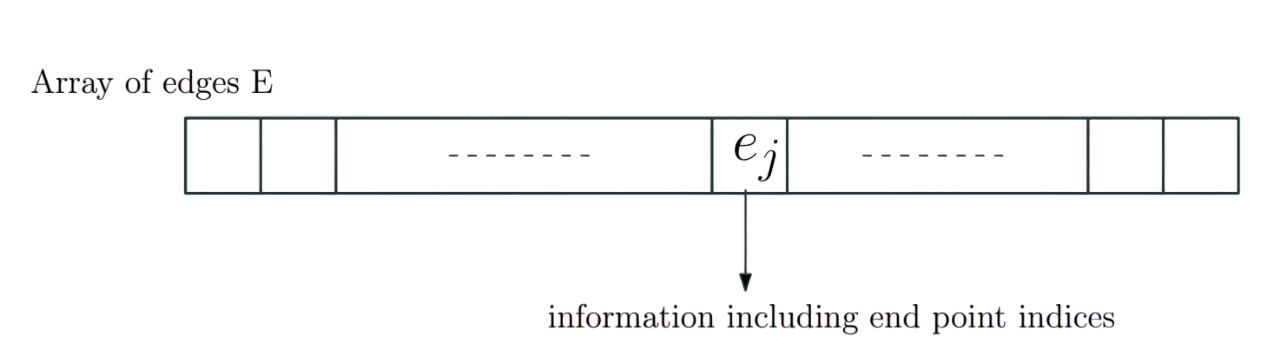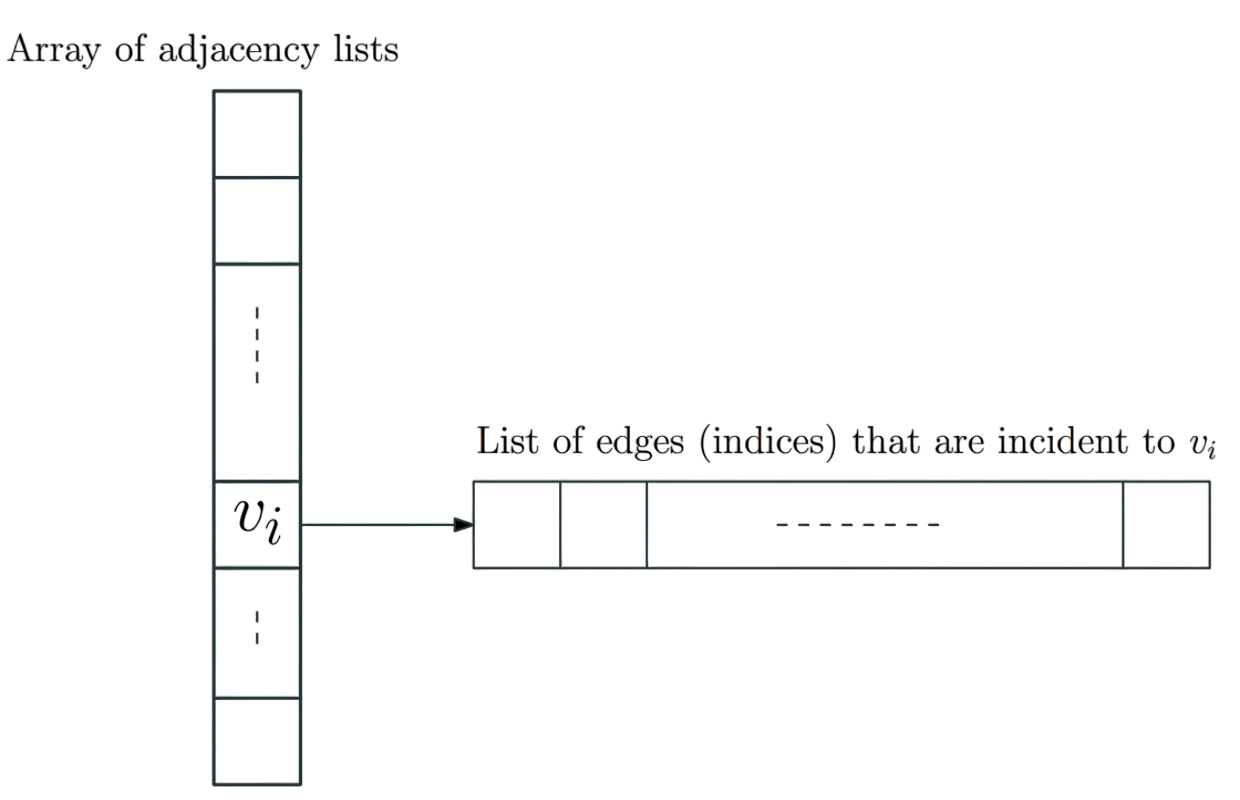• Edges are explicitly represented/numbered. Scanning/processing all edges easy to do.
• Representation easily supports multigraphs including self-loops.
• Explicit numbering of vertices and edges allows use of arrays: O(1)-time operations are easy to understand.
• Can also implement via pointer based lists for certain dynamic graph settings.
##### Connectivity

Given a graph G = (V, E):

• path: sequence of distinct vertices $v_1$ , $v_2$ ,…, $v_k$ such that $v_i$ $v_{i+1}$ $\in$ E for 1 $\le$ i $\le$ k-1. The length of the path is k-1 (the number of edges in the path) and the path is from v$_1$ to v$_k$. Note: a single vertex u is a path of length 0.
• cycle: sequence of distinct vertices $v_1$, $v_2$,…, $v_k$ such that {$v_i$, $v_{i+1}$} $\in$ E for 1 $\le$ i $\le$ k1 and {$v_1$, $v_k$} $\in$ E. Single vertex not a cycle according to this defnition.
• Caveat: Some times people use the term cycle to also allow vertices to be repeated; we will use the term tour.
• A vertex u is connected to v if there is a path from u to v.
• The connected component of u, con(u), is the set of all vertices connected to u.
• In undirected graphs, connectivity is a refexive, symmetric, and transitive relation. Connected components are the equivalence classes.
• Graph is connected if there is only one connected component.In undirected graphs, connectivity is a refexive, symmetric, and transitive relation. Connected components are the equivalence classes.
• Graph is connected if there is only one connected component. Example: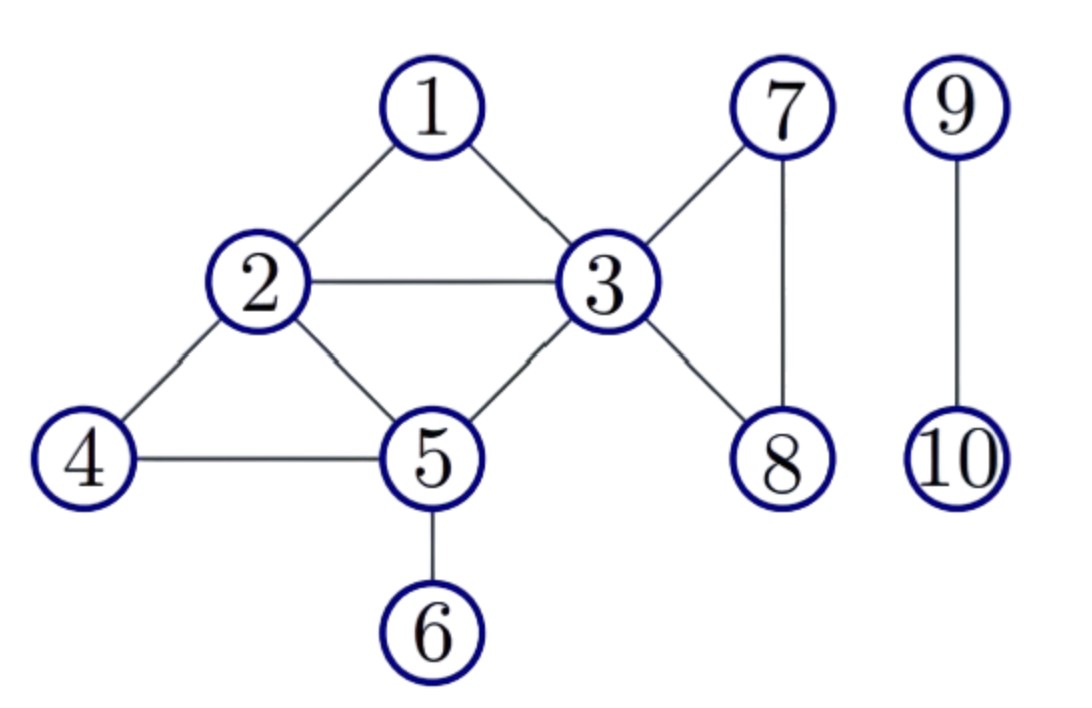##### Basic Graph Search in Undirected Graphs:
 Given G = (V,E) and vertex u $\in$ V. Let n = V . Then:
Explore(G,u):
Visited[1 . . n] = FALSE
//ToExplore, S: Lists
Add u to ToExplore and to S
Visited[u] = TRUE
while (ToExplore is non-empty) do
Remove node x from ToExplore
for each edge xy in Adj(x) do
if (Visited[y] = FALSE)
Visited[y] = TRUE
Output S


Running time : O(n+m)

• Special cases :
• Breadth First Search (BFS): use queue data structure to implementing the list.
• Depth First Search (DFS): use stack data structure to implement the list.
• Search tree: One can create a natural search tree T rooted at u during search.
Explore(G,u):
array Visited[1..n]
Initialize: Visited[i] = FALSE for i = 1,..., n
List: ToExplore, S
Add u to ToExplore and to S, Visited[u] = TRUE
Make tree T with root as u
while (ToExplore is non-empty) do
Remove node x from ToExplore
for each edge (x, y) in Adj(x) do
if (Visited[y] = FALSE)
Visited[y] = TRUE
Add y to T with x as its parent
Output S


#### II. Directed graphs

##### Definition
• A directed graph G = (V, E) consists of
• set of vertices/nodes V and
• a set of edges/arcs E \subseteq V x V.
• An edge is an ordered pair of vertices. (u, v) diferent from (v, u).
• Examples:
• Road networks with one-way streets.
• Web-link graph: vertices are web-pages and there is an edge from page p1 to page p2 if p1 has a link to p2.
• Web graphs used by Google with PageRank algorithm to rank pages.
• Dependency graphs in variety of applications: link from x to y if y depends on x. Make fles for compiling programs.
• Program Analysis: functions/procedures are vertices and there is an edge from x to y if x calls y.
##### Representation

Graph G = (V, E) with n vertices and m edges:

• Adjacency Matrix: n x n asymmetric matrix A. A[u, v] = 1 if (u, v) $\in$ E and A[u, v] = 0 if (u, v) $\notin$ E. A[u, v] is not same as A[v, u].
• Adjacency Lists: for each node u, Out(u) (also referred to as Adj(u)) and In(u) store out-going edges and in-coming edges from u.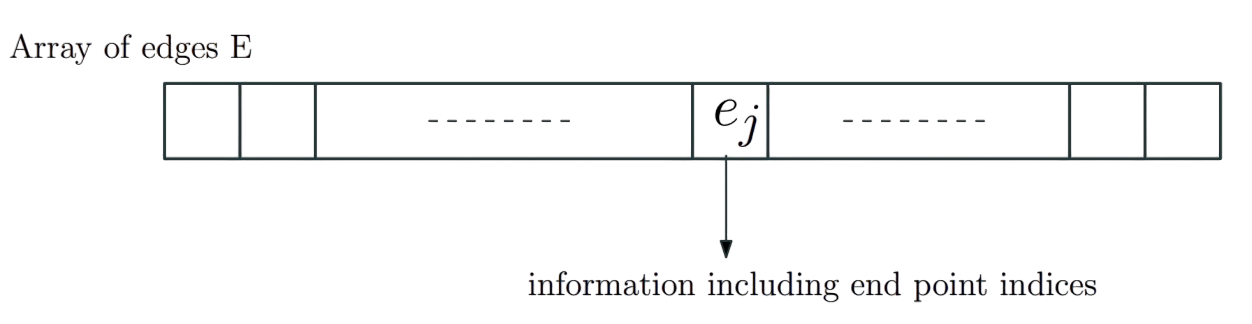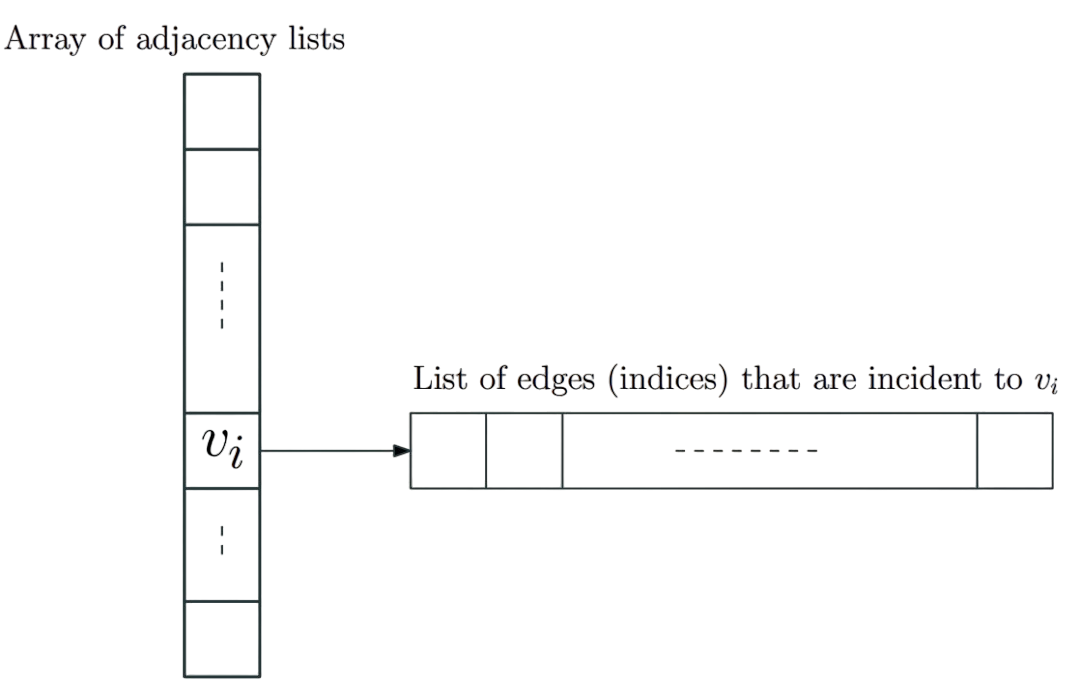##### Directed Connectivity

Given a graph G = (V, E):

• A (directed) path is a sequence of distinct vertices $v_1$ , $v_2$ ,…, $v_k$ such that ($v_i$, $v_{i+1}$) $\in$ E for 1 $\le$ i $\le$ k-1. The length of the path is k-1 and the path is from $v_1$ to $v_k$. By convention, a single node u is a path of length 0.
• A cycle is a sequence of distinct vertices $v_1$ , $v_2$ ,…, $v_k$ such that ($v_i$, $v_{i+1}$) $\in$ E for 1 $\le$ i $\le$ k-1 and ($v_k$, $v_1$) $\in$ E. By convention, a single node u is not a cycle.
• A vertex u can reach v if there is a path from u to v. Alternatively v can be reached from u
• Let rch(u) be the set of all vertices reachable from u.

Example of Asymmetricity: D can reach B but B cannot reach D.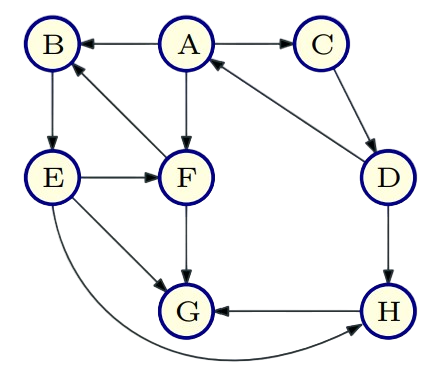##### Strong Connected Components
• Defnition: Given a directed graph G, u is strongly connected to v if u can reach v and v can reach u. In other words v $\in$ rch(u) and u $\in$ rch(v).
• Define relation C where uCv if u is (strongly) connected to v.
• Proposition: C is an equivalence relation, that is refexive, symmetric and transitive.
• Equivalence classes of C: strong connected components of G. They partition the vertices of G.
• SCC(u): strongly connected component containing u.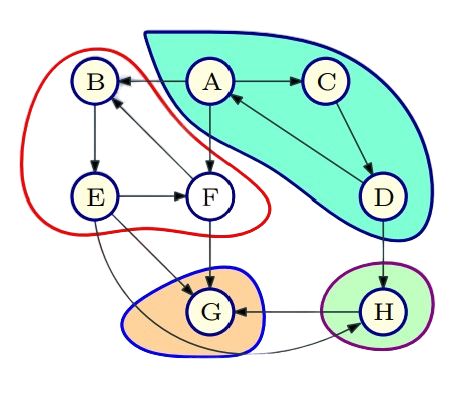##### Basic Graph Search in Directed Graphs

Given G = (V,E) and vertex u $\in$ V. Let n = |V|. Then:

Explore(G,u):
array Visited[1..n]
Initialize: Set Visited[i] = FALSE for 1 <= i <= n
List: ToExplore, S
Add u to ToExplore and to S, Visited[u] = TRUE
Make tree T with root as u
while (ToExplore is non-empty) do
Remove node x from ToExplore
for each edge (x, y) in Adj(x) do
if (Visited[y] = FALSE)
Visited[y] = TRUE
Add y to T with edge (x, y)
Output S


Example - rch(B) :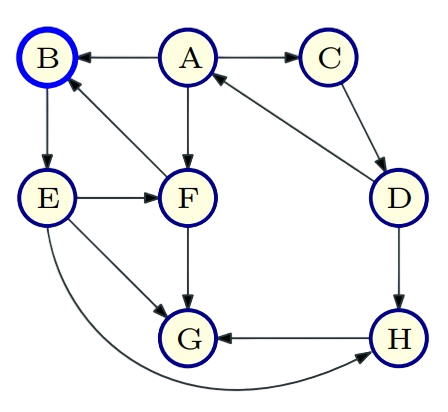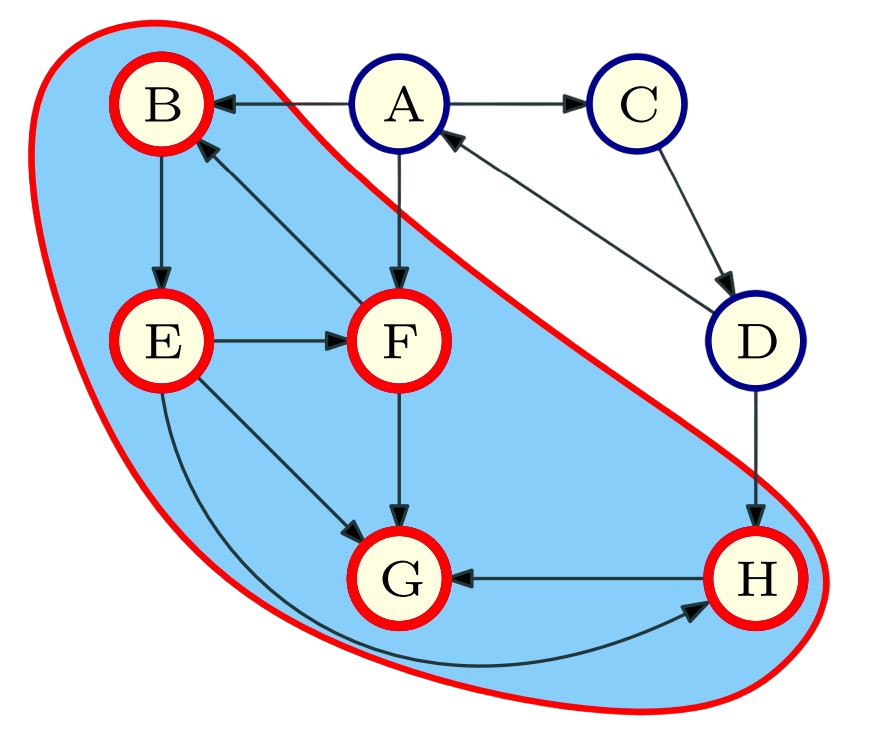##### Basic Graph Search Properties
• Proposition : Explore(G, u) terminates with S = rch(u).
• Proof Sketch.
• Once Visited[i] is set to TRUE it never changes. Hence a node is added only once to ToExplore. Thus algorithm terminates in at most n iterations of while loop.
• By induction on iterations, can show v ∈ S ⇒ v ∈ rch(u)
• Since each node v ∈ S was in ToExplore and was explored, no edges in G leave S. Hence no node in V − S is in rch(u).
• Caveat: In directed graphs edges can enter S.
• Thus S = rch(u) at termination

• Textbooks
• Erickson, Jeff. Algorithms
• Skiena, Steven. The Algorithms Design Manual
• Chapter 7.5 - Traversing a Graph
• Sedgewick, Robert and Wayne, Kevin. Algorithms (Forth Edition)
• Chapter 4 - Graphs
• Cormen, Thomas, et al. Algorithms (Forth Edition)
• Chapter 20 - Elementary Graph Algorithms
• Sariel’s Lecture 15